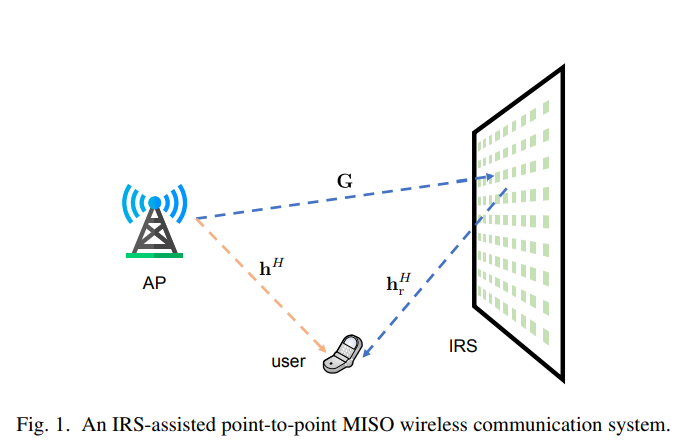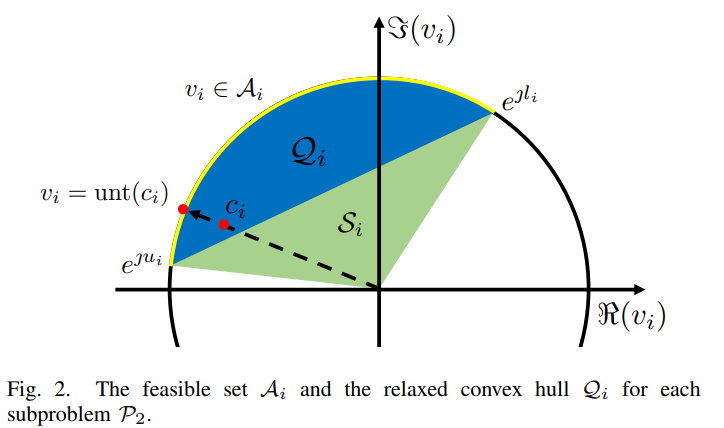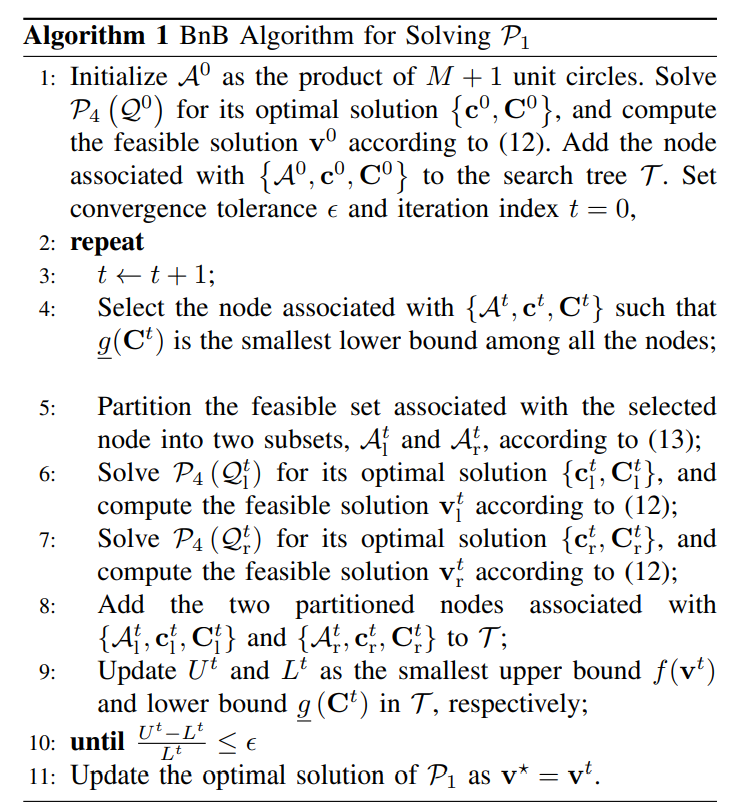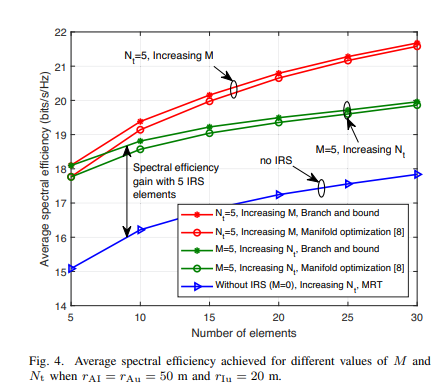# 基于智能反射面的MISO通信的最优波束赋形

### 文章目录

《Optimal Beamforming for MISO Communications via Intelligent Reflecting Surfaces》

# 系统模型$y=\left(\mathbf{h}_{\mathrm{r}}^{H} \mathbf{\Phi} \mathbf{G}+\mathbf{h}^{H}\right) \mathbf{f} x+n$

# 问题描述

$R=\log _{2}\left(1+\frac{\left|\left(\mathbf{h}_{\mathrm{r}}^{H} \mathbf{\Phi} \mathbf{G}+\mathbf{h}^{H}\right) \mathbf{f}\right|^{2}}{\sigma^{2}}\right)$

$\begin{array}{ll}{\underset{\mathbf{f}, \mathbf{\Phi}}{\operatorname{maximize}}} & {\left|\left(\mathbf{h}_{\mathbf{r}}^{H} \mathbf{\Phi} \mathbf{G}+\mathbf{h}^{H}\right) \mathbf{f}\right|^{2}} \\ {\text { subject to }} & {\|\mathbf{f}\|_{2}^{2} \leq P} \\ {} & {\mathbf{\Phi}=\operatorname{diag}\left(e^{\jmath \theta_{1}}, e^{\jmath \theta_{2}}, \ldots, e^{\jmath^{g} M}\right)}\end{array}$

\begin{aligned} &\underset{\mathbf{v} \in \mathbb{C}^{M+1}}{\operatorname{minimize}} f(\mathbf{v})=\mathbf{v}^{H} \mathbf{R} \mathbf{v}\\ &\text { subject to } \quad\left|v_{i}\right|=1, \quad i=1,2, \dots, M+1 \end{aligned}

$\mathbf{R}=-\left[\begin{array}{cc}{\operatorname{diag}\left(\mathbf{h}_{\mathrm{r}}^{H}\right) \mathrm{G} \mathbf{G}^{H} \operatorname{diag}\left(\mathbf{h}_{\mathrm{r}}\right)} & {\operatorname{diag}\left(\mathbf{h}_{\mathrm{r}}^{H}\right) \mathrm{Gh}} \\ {\mathbf{h}^{H} \mathbf{G}^{H} \operatorname{diag}\left(\mathbf{h}_{\mathrm{r}}\right)} & {0}\end{array}\right]$

$\mathbf{f}=\sqrt{P} \frac{\mathbf{G}^{H} \operatorname{diag}\left(\mathbf{h}_{\mathbf{r}}\right) \mathbf{x}+\mathbf{h}}{\left\|\mathbf{G}^{H} \operatorname{diag}\left(\mathbf{h}_{\mathbf{r}}\right) \mathbf{x}+\mathbf{h}\right\|_{2}}$

• 对于问题$\mathcal{P}_{1}$$\mathbf{R}$非正定矩阵，且约束条件含有恒模约束，这些都使得问题高度非凸。总之，$\mathcal{P}_{1}$是一个搜索维度为$M+1$的NP-hard问题。
• 采用半正定松弛（SDR）的方法可以处理问题$\mathcal{P}_{1}$，其中辅助变量$\mathbf{V}=\mathbf{v} \mathbf{v}^{H}$被引入将$\mathcal{P}_{1}$重新表示为一个带有额外秩1约束的半正定规划（SDP）问题。然后，释放秩1约束，使用凸优化的方法就可以很好地求解SDP问题，但并不能保证所得到$\mathbf{V}$满足秩1条件，采用高斯随机方法可以保证其渐进最优。因此，SDR方法提供了$\mathbf{v}$的一个近似解.
• 采用流行优化的方法可以获得问题$\mathcal{P}_{1}$的一个局部最优解.，即使如此，其性能十分优秀。在这篇论文中，作者提出了分支界定法来得到$\mathcal{P}_{1}$全局最优解并研究了流行优化方法的最优度。

# 分支界定算法

## 下界与上界

\begin{aligned} &\underset{\mathbf{v} \in \mathbb{C}^{M+1}}{\operatorname{minimize}} f(\mathbf{v})=\mathbf{v}^{H} \mathbf{R} \mathbf{v}\\ &\text { subject to } v_{i} \in \mathcal{A}_{i}, \quad \forall i \end{aligned}

$\begin{array}{cl} {\underset{\mathbf{v}, \mathbf{V} \succeq \mathbf{0}}{\operatorname{minimize}}} & {\underline{g}(\mathbf{V})=\operatorname{tr}(\mathbf{R} \mathbf{V})} \\ {\text { subject to }} & {v_{i} \in \mathcal{A}_{i}, \quad \forall i} \\ {} & {\operatorname{Diag}(\mathbf{V})=\mathbf{1}_{M+1}} \\ {} & {\mathbf{V}=\mathbf{v} \mathbf{v}^{H}} \end{array}$$\begin{array}{cl} {\underset{\mathbf{c}, \mathbf{C} \succeq \mathbf{0}}{\operatorname{minimize}}} & {\underline{g}(\mathbf{C})=\operatorname{tr}(\mathbf{R} \mathbf{C})} \\ {\text { subject to }} & {c_{i} \in \mathcal{Q}_{i}, \quad \forall i} \\ {} & {\operatorname{Diag}(\mathbf{C})=\mathbf{1}_{M+1}} \\ {} & {\mathbf{C} \succeq \mathbf{c} \mathbf{c}^{H}} \end{array}$

$\Re\left(\mathbf{a}^{*} \circ \mathbf{c}\right) \geq \cos \left(\frac{\mathbf{u}-1}{2}\right)$

$\left[\begin{array}{cc}{1} & {\mathbf{c}^{H}} \\ {\mathbf{c}} & {\mathbf{C}}\end{array}\right] \succeq 0$

## 节点选择和剪枝规则

$i^{\star}=\arg \max _{i} \quad\left|c_{i}-v_{i}\right|$# 仿真分析• 对于频谱效率的提升，增加IRS可配置移相器的数目比增加AP天线数目更加有效，并且随着天线规模的数目增多，其带来的能源开销相较于无源的IRS是十分明显的。
• BnB算法可以作为现存次优算法的一个性能基准。
• MO算法是设计IRS协助MISO通信的一个有效算法，能够很好地逼近BnB算法。

©️2019 CSDN 皮肤主题: 大白 设计师: CSDN官方博客